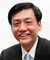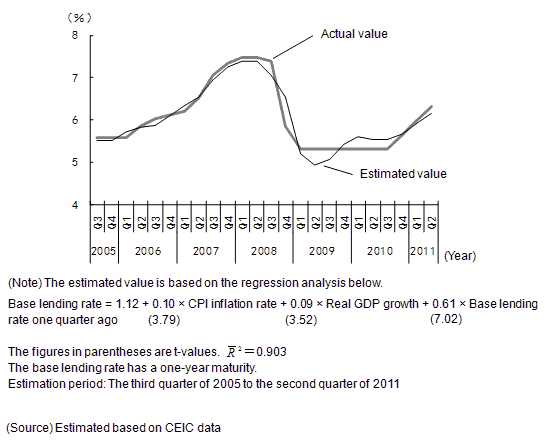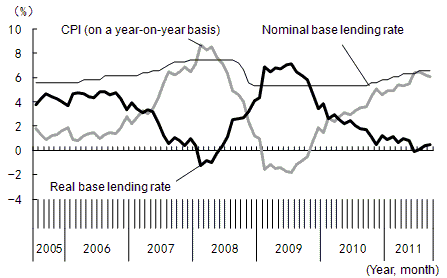China in Transition

# Determinants of the Policy Interest Rate in ChinaImplications of the Taylor RuleChi Hung KWAN
Consulting Fellow, RIETI

China has been grappling with rising inflation since last year, with the consumer price index (CPI) inflation rate climbing to 6.5% in July 2011, the highest in 37 months. To control inflation, the authorities have changed their stance on monetary policy from easing to tightening, raising the benchmark one-year base lending rate five times, for a total of 1.25 percentage points, since October 2010. As interest rate behavior has significant implications for the future of the economy, its direction attracts attention. Although the inflation rate measured in terms of the Consumer Price Index (CPI) declined slightly from July to 6.2% in August, market fears of a rate hike have not been completely dispelled. In this column, the possibility of a further rate hike will be discussed by applying the Taylor rule—according to which the authorities should adjust monetary policy in response to inflation and the level of the economic activity—to the analysis of monetary policy in China, regarding the rule as a policy reaction function of the monetary authorities.

## What is the Taylor rule?

The Taylor rule was put forward by John B. Taylor, a former Under Secretary of the U.S. Treasury (2001-2005) and current professor of economics at Stanford University, as a guide to formulating monetary policy. The rule proposes that the policy interest rate should be determined in response to the divergence of actual inflation from the target long-term inflation and divergence in the level of economic activity from its equilibrium value (as measured by the GDP gap). The monetary authorities should raise the policy interest rate when the actual inflation rate exceeds the target inflation rate or when real GDP exceeds potential GDP, and should lower it when the opposite applies. The optimal level of the policy interest rate under the Taylor rule is obtained by the following equation:

Optimal level of policy interest rate = Actual inflation rate + Equilibrium real interest rate + 0.5 x (Actual inflation rate - Target inflation rate) + 0.5 x (GDP gap) (Note 1).

Using the example of the United States, if we assume that the equilibrium real interest rate is 2% and the target inflation rate is 2%, then the optimal level of the federal funds (FF) rate, which is the policy interest rate, can be obtained by the following equation:

 Optimal level of the FF rate = Actual inflation rate + 2% + 0.5 x (Actual inflation rate - 2%) + 0.5 x (GDP gap) = 1.5 x (Actual inflation rate) + 0.5 x (GDP gap) + 1%

According to the Taylor rule, the Fed should raise the FF rate by 1.5 percentage points in response to a one-percentage point rise in the inflation rate and raise the FF rate by 0.5 percentage points with a one-percentage point expansion of the GDP gap. The idea that the policy interest rate must be raised by more than the rise in the inflation rate in order to stabilize the macro economy is called the Taylor principle.

As noted, the Taylor rule was originally developed to determine the optimal level of the policy interest rate. However, the aspect of a policy reaction function of monetary authorities to macroeconomic changes has come to be emphasized, as the rule has also been found to be effective in explaining changes in the policy interest rate in the United States. In this case, the elasticities of the policy interest rate to changes in the inflation rate (or more precisely, the difference between the actual inflation rate and the target inflation rate) and changes in the GDP gap are to be confirmed by empirical analysis, and it is not assumed in the analysis that these elasticities will match their optimal values (1.5 times and 0.5 times to changes in the inflation rate and the GDP gap, respectively) originally proposed by the Taylor rule.

## Applying the Taylor rule to China

Regarding the Taylor rule as a policy reaction function, determinants of the monetary policy in China will be analyzed using the statistical method of regression. The period subject to analysis is from the third quarter of 2005, starting from July, when China moved from the dollar peg system to the managed floating rate system, to the second quarter of 2011.

In the case of China, the benchmark of the policy interest rate, which is the explained variable in our regression analysis, is the one-year base lending rate of financial institutions. Meanwhile, what corresponds to the inflation rate, which is the first explanatory variable, is the CPI rate on a year-on-year basis. As for the second explanatory variable, since there are no official statistics corresponding to the GDP gap, real GDP growth is used as a proxy variable (Note 2). In addition, given the inertia of monetary policy, the base lending rate one quarter ago is added to the equation as the third explanatory variable (Note 3). The result of our estimation shows that the monetary authorities respond to a one-percentage point rise in the inflation rate and a one-percentage point rise in the growth rate by raising the interest rate by 0.10 percentage points and 0.09 percentage points, respectively (Figure 1).

Figure 1: Changes in the One-Year Base Lending Rate: Estimated Value vs. Actual ValueHowever, it is contrary to the Taylor principle for the authorities to raise (lower) the policy interest rate by only 0.10 percentage points when inflation rises (falls) by one-percentage point. Reflecting this, the real interest rate in China is inversely correlated with the inflation rate, and it is obvious that interest rate policy is not working sufficiently to stabilize the economy (Figure 2).

Figure 2: Real Base Lending Rate Inversely Correlated with the Inflation Rate(Note) The base lending rate has a one-year maturity. Real base lending rate = Nominal base lending rate - CPI (on a year-on-year basis)
(Source) Estimated based on CEIC data

## Will a further rate hike be implemented?

Analysis based on the Taylor rule is useful not only for explaining interest rate behavior in the past, but also for predicting interest rates in the future. Based on the regression analysis above, the theoretical value of the one-year base lending rate is estimated to be 6.39%, given the assumption that real GDP growth is 9.2% and the inflation rate is 6.2% as of the third quarter of 2011. However, the actual base lending rate after the rate hike was implemented on July 7, 2011 is 6.56%, already exceeding the theoretical value. In addition, given that inflation declined from 6.5% in July to 6.2% in August and that both inflation and growth are expected to slow down going forward, the possibility of a further rate hike appears to be limited.

Footnote(s)

Note 1: The GDP gap is calculated by subtracting the potential level of real GDP from actual real GDP, thus, the larger the value, the more overheated the economy.

Note 2: Strictly speaking, the difference between real GDP growth and the potential growth should be used as a proxy variable for the GDP gap. For simplification, however, only the real GDP growth is added to the estimation equation, assuming that potential growth stays constant.

Note 3: For simplification, the equilibrium real interest rate and the target inflation rate, which are part of the Taylor rule, are assumed to stay constant and excluded from the estimation equation.

Related article

September 30, 2011

## Article(s) by this author

• ### China's Economy to Slow Down in 2022—The Prospects of the Housing Market and COVID Control in the Spotlight

March 10, 2022［China in Transition］

• ### China Aims for "Common Prosperity"—Increasing rural incomes and secondary distribution reform as the key

March 8, 2022［China in Transition］

• ### Tightening Regulation of Private Enterprises in China—Focusing on the Cases of Ant Group and Didi

January 17, 2022［China in Transition］

• ### China Aims for Carbon Neutrality—Decarbonization of Energy and Industrial Structures is the Key

October 6, 2021［China in Transition］

• ### Challenges for the Chinese Economy as Viewed through the 2020 Population Census—Focusing on a Declining Labor Force and Inter-Regional Migration

July 20, 2021［China in Transition］